Next: The Complete Quadrangle Up: Harmonic Ratios and Involutions Previous: Harmonic Ratios and Involutions

## Definition

Let A, B, C, D be four points on a line with cross ratio k. From the definition of the cross ratio, it follows that the 4!=24possible permutations of the points yield 6 different (but functionally equivalent) cross ratios: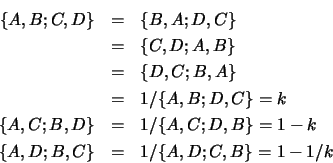In some symmetrical cases, the six values reduce to three or even two. When two points coincide, the possible values are 0, 1 and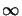. With certain symmetrical configurations of complex points the possible values are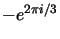and. Finally, when k=-1the possible values are -1, 1/2 and 2. The Ancient Greek mathematicians called this a harmonic configuration. The couples (A, B) and (C, D) are said to be harmonic pairs, and we have the following equality: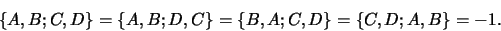Hence, the harmonic cross ratio is invariant under interchange of points in each couple and also (as usual) under interchange of couples, so the couples can be considered to be unordered. Given (A, B), D is said to be conjugate to C if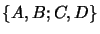forms a harmonic configuration.

Exercise 3.5   :  If C is at infinity, where is its harmonic conjugate?

The previous exercise explains why the harmonic conjugate is considered to be a projective extension of the notion of an affine mid-point.Next: The Complete Quadrangle Up: Harmonic Ratios and Involutions Previous: Harmonic Ratios and Involutions
Bill Triggs
1998-11-13